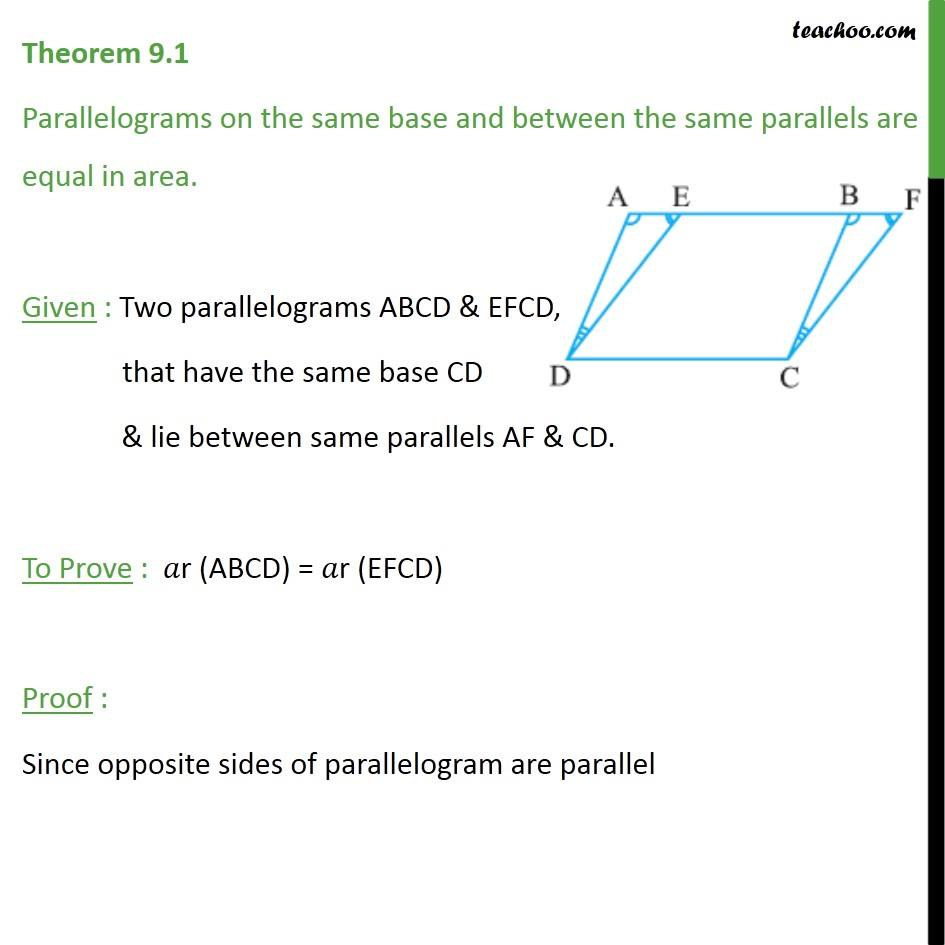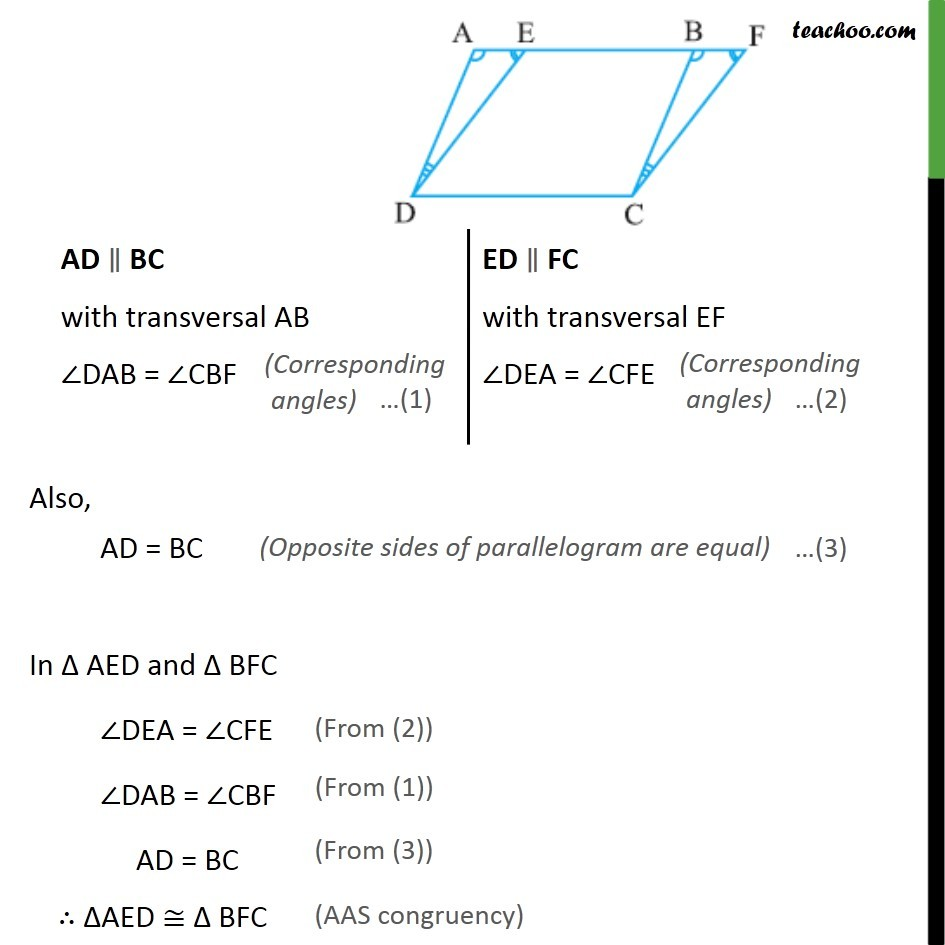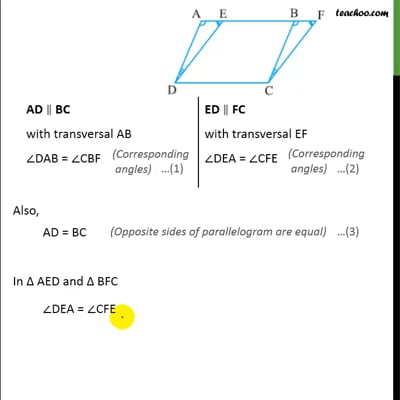Theorems

Chapter 9 Class 9 - Areas of Parallelograms and Triangles [Deleted]
Serial order wiseThis video is only available for Teachoo black users

Solve all your doubts with Teachoo Black (new monthly pack available now!)

### Transcript

Theorem 9.1 Parallelograms on the same base and between the same parallels are equal in area. Given : Two parallelograms ABCD & EFCD, that have the same base CD & lie between same parallels AF & CD. To Prove : r (ABCD) = r (EFCD) Proof : Since opposite sides of parallelogram are parallel Also, AD = BC In AED and BFC DAB = CBF DEA = CFE AD = BC AED BFC AED BFC Hence, r ( AED) = r ( BFC) Now, r (ABCD) = r ( ADE) + r(EBCD) = r ( BFC) + r (EBCD) = r ( EFCD) Hence, proved